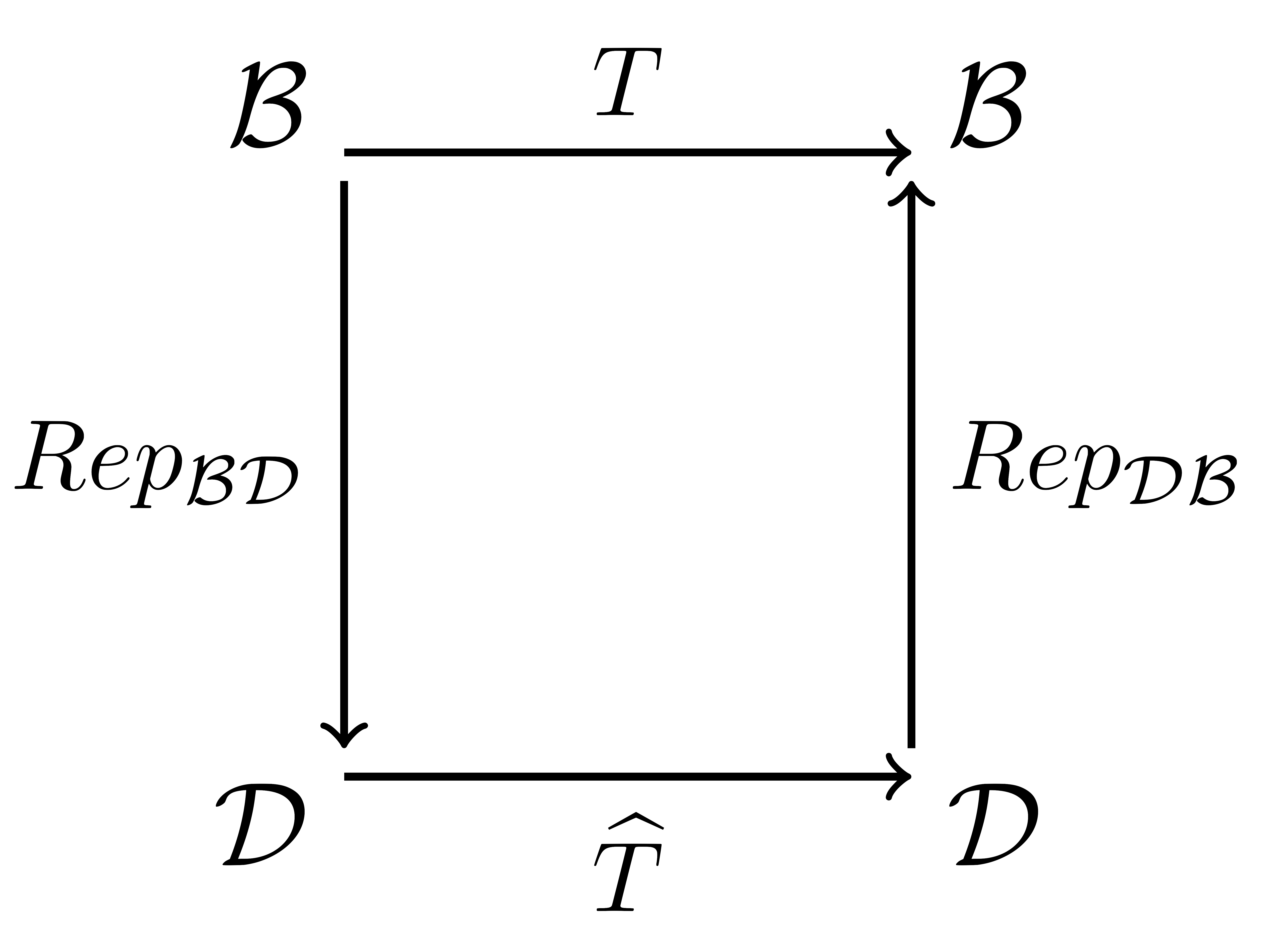## Section4.2Matrix Equivalence and Similarity

### Subsection4.2.1Change of Bases and Transformations

Recall that if $\mathcal{B}$ is one basis for a vector space and $\mathcal{D}$ is another, then the transformations $Rep_{\mathcal{B}\mathcal{D}}$ and $Rep_{\mathcal{D}\mathcal{B}}$ change coordinates back and forth between them, and that $(Rep_{\mathcal{D}\mathcal{B}})^{-1}=Rep_{\mathcal{B}\mathcal{D}}\text{.}$ (See also Figure 3.4.5 and Figure 3.4.6.)

If $T$ is a transformation of the vector space in terms of the basis $\mathcal{B}\text{,}$ then

\begin{align*} \widehat{T}\amp=Rep_{\mathcal{D}\mathcal{B}}\circ T\circ Rep_{\mathcal{B}\mathcal{D}}\\ \amp=Rep_{\mathcal{D}\mathcal{B}}\circ T\circ (Rep_{\mathcal{D}\mathcal{B}})^{-1}, \end{align*}

is the same transformation except in terms of $\mathcal{D}\text{.}$  1 Why is the composition of the transformations written right to left? When this situation exists we say that the transformations $T$ and $\widehat{T}$ are similar (or in some settings we say they are conjugate).In your text (map representations, p.259 and similarity, p.388) you will see this written something like:

\begin{equation*} T=Rep_{\mathcal{B}\mathcal{B}}\left(T\right) \end{equation*}

and

\begin{align*} \widehat{T}\amp=Rep_{\mathcal{D}\mathcal{D}}\left(T\right)\\ \amp=Rep_{\mathcal{D}\mathcal{B}}\circ T\circ (Rep_{\mathcal{D}\mathcal{B}})^{-1}\\ \amp=Rep_{\mathcal{D}\mathcal{B}}\circ Rep_{\mathcal{B}\mathcal{B}}\left(T\right)\circ (Rep_{\mathcal{D}\mathcal{B}})^{-1}. \end{align*}

### Subsection4.2.2Similar Matrices

###### Definition4.2.2. Similar Matrices.

Two matrices $A$ and $B$ are said to be similar if there exists an invertible (non-singular) matrix $P$ such that

\begin{equation*} B=P\, A\, P^{-1}. \end{equation*}

More generally, we say that two elements related in this way are conjugates.

###### Investigation4.2.1. A Basic Example.

Suppose we have two matrices

\begin{equation*} A= \left( \begin{array}{rr} 1\amp 3 \\ -1 \amp-2\\ \end{array} \right),\ \mbox{and}\ B= \left( \begin{array}{rr} -10 \amp 7 \\ -13 \amp 9 \end{array} \right) \end{equation*}

and we want to see if they are similar. By definition this means we need to find $P$ such that

\begin{equation*} A=P\, B\, P^-1 \end{equation*}

or equivalently

\begin{equation*} A\, P=P\, B. \end{equation*}

Let

\begin{equation*} P= \left( \begin{array}{rr} p_1 \amp p_2\\ p_3 \amp p_4 \end{array} \right) \end{equation*}

so that the equation above becomes

\begin{equation*} \left( \begin{array}{rr} p_1+3p_3\amp p_2+3p_4 \\ -p_1-2p_3 \amp -p_2-2p_4\\ \end{array} \right)= \left( \begin{array}{rr} -10p_1-13p_2\amp 7p_1+9p_2 \\ -10p_3-13p_4\amp 7p_3+9p_4 \\ \end{array} \right) \end{equation*}

leaving us with four equations and four unknowns. Here we write it as a homogeneous system:

\begin{align*} 11p_1+13p_2+3p_3\amp = 0\\ 7p_1+8p_2-3p_4\amp = 0\\ p_1-8p_3-13p_4\amp = 0\\ p_2+7p_3+11p_4\amp = 0 \end{align*}

or as a matrix equation

\begin{equation*} \left( \begin{array}{rrrr|r} 11 \amp 13 \amp 3 \amp 0 \amp 0\\ 7 \amp 8 \amp 0 \amp -3 \amp 0\\ 1 \amp 0 \amp -8 \amp -13 \amp 0\\ 0 \amp 1 \amp 7 \amp 11 \amp 0\\ \end{array} \right). \end{equation*}

With a little work this reduces to

\begin{equation*} \left(\begin{array}{rrrr|r} 1 \amp 0 \amp -8 \amp -13 \amp 0\\ 0 \amp 1 \amp 7 \amp 11 \amp 0\\ 0 \amp 0 \amp 0 \amp 0 \amp 0\\ 0 \amp 0 \amp 0 \amp 0 \amp 0 \end{array}\right). \end{equation*}

So if we let $p_3=-1$ and $p_4=1$ we get $p_1=5$ and $p_2=-4\text{,}$ and

\begin{equation*} P= \left( \begin{array}{rr} 5 \amp -4\\ 1 \amp -1 \end{array} \right). \end{equation*}

Note that since $p_3$ and $p_4$ are free variables there are in fact infinitely many solutions. Finally, we will see in Subsection 4.3.1 that there is an easier way.

Here are some basic key observations: